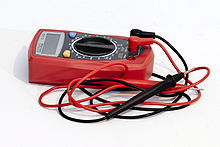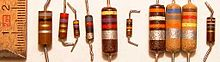# Ohm

﻿
OhmA multimeter can be used to measure resistance in ohms. It can also be used to measure capacitance, voltage, current, and other electrical characteristics.

The ohm (symbol: Ω) is the SI unit of electrical resistance, named after German physicist Georg Simon Ohm.

## DefinitionSeveral resistors. Their resistance, in ohms, is marked using a color code.

The ohm is defined as a resistance between two points of a conductor when a constant potential difference of 1 volt, applied to these points, produces in the conductor a current of 1 ampere, the conductor not being the seat of any electromotive force.$\Omega = \dfrac{\mbox{V}}{\mbox{A}} = \dfrac{\mbox{m}^2 \cdot \mbox{kg}}{\mbox{s} \cdot \mbox{C}^2} = \dfrac{\mbox{J}}{\mbox{s} \cdot \mbox{A}^2}=\dfrac{\mbox{kg}\cdot\mbox{m}^2}{\mbox{s}^3 \cdot \mbox{A}^2}= \dfrac{\mbox{J} \cdot \mbox{s}}{\mbox{C}^2}$

In many cases the resistance of a conductor in ohms is approximately constant within a certain range of voltages, temperatures, and other parameters; one speaks of linear resistors. In other cases resistance varies (e.g., thermistors).

Commonly used multiples and submultiples in electrical and electronic usage are the milliohm, ohm, kilohm, megohm, and gigaohm.

In alternating current circuits, electrical impedance is also measured in ohms.

## Conversions

The SI unit of electrical conductance is the siemens, also known as the mho (ohm spelled backwards, symbol is ℧); it is the reciprocal of resistance in ohms.

## Power as a function of resistance

The power dissipated by a linear resistor may be calculated from its resistance, and voltage or current. The formula is a combination of Ohm's law and Joule's laws:$P=V\cdot I =\frac{V^2}{R} = I^2\cdot R$

where P is the power in watts, R the resistance in ohms, V the voltage across the resistor, and I the current through it.

This formula is applicable to devices whose resistance varies with current.

## Use of the Ω symbol in electronic documents

Care should be taken when preparing documents (including HTML documents) that make use of the symbol Ω. Some document editing software will attempt to use the symbol typeface to render the character. Where the font is not supported, a W is displayed instead (a "10 W" resistor instead of a "10 Ω" resistor, for instance). As this represents the SI unit of power, not resistance, this can lead to confusion.

Unicode encodes an ohm symbol (U+2126 , Ω) distinct from Greek omega among letterlike symbols, but it is only included for backwards compatibility and the Greek uppercase omega character (U+03A9 Ω (HTML: &#937; &Omega;), Ω) is preferred.. Many text editors allow the use of ALT 234 to produce the Ω symbol.

Wikimedia Foundation. 2010.

### Look at other dictionaries:

• ohm — ohm …   Dictionnaire des rimes

• ohm — [ om ] n. m. • 1867; nom d un physicien all. ♦ Métrol. Unité de mesure de résistance électrique (symb.Ω). L ohm, ses multiples et ses sous multiples (kilohm, mégohm, microhm...) sont conservés à l aide de résistances étalons faites de fil de… …   Encyclopédie Universelle

• ohm — OHM, ohmi, s.m. Unitate de măsură a rezistenţei electrice, în sistemul de unităţi metru kilogram secundă amper, egală cu rezistenţa pe care o opune un conductor electric străbătut de un curent de un amper la tensiunea de un volt. [pr.: om] – Din… …   Dicționar Român

• Ohm — /ohm/, n. Georg Simon, /gay awrddk zee mawn/ 1787 1854, German physicist. * * * ▪ unit of energy measurement       abbreviation Ω, unit of electrical resistance in the metre kilogram second system, named in honour of the 19th century German… …   Universalium

• ohm — òhm s.m.inv. TS fis., elettr. nel Sistema Internazionale, unità di misura della resistenza elettrica (simb. Ω) {{line}} {{/line}} DATA: 1892. ETIMO: da Ohm, nome del fisico tedesco Georg Simon Ohm (1787 1854). POLIREMATICHE: ohm acustico: loc.s.m …   Dizionario italiano

• Ohm —    , OHM S LAW    Georg Simon Ohm (1787 1854), was born in Erlangen, Bavaria, Germany, to a family of locksmiths.    Ohm received a doctorate in physics when he was twenty two and then accepted a position teaching mathematics at the Jesuit… …   Dictionary of eponyms

• Ohm — ([=o]m), n. [So called from the German electrician, G. S. Ohm.] (Elec.) The standard unit in the measure of electrical resistance, being the resistance of a circuit in which a potential difference of one volt produces a current of one amp[ e]re.… …   The Collaborative International Dictionary of English

• Ohm — Ohm, vorzugsweise ein Weinmaß, das aber in den meisten Ländern verschieden ist. In Hamburg z. B. rechnet man auf 1 Ohm 5 Eimer oder 80 Kannen, in Hannover 60 Kannen, eben so in Schweden, in Würtemberg 160 Maß, in Baden 100 Maß etc. Fast überall… …   Damen Conversations Lexikon

• ohm — [əum US oum] n [Date: 1800 1900; Origin: Georg Simon Ohm (1787 1854), German scientist] a unit for measuring electrical ↑resistance …   Dictionary of contemporary English

• ohm — (n.) unit of electrical resistance, 1867, in recognition of German physicist Georg S. Ohm (1789 1854), who determined the law of the flow of electricity. Originally proposed as ohma (1861) as a unit of voltage. Related: ohmage; ohmic; ohmeter …   Etymology dictionary

• ohm — (izg. ȏm) m DEFINICIJA izvedena SI jedinica električnog otpora (simbol Ω); otpor između dvije točke vodiča iznosi 1 Ω ako pod utjecajem potencijalne razlike (između tih točaka) od jednog volta vodičem teče struja od jednog ampera (Ω = V/A)… …   Hrvatski jezični portal